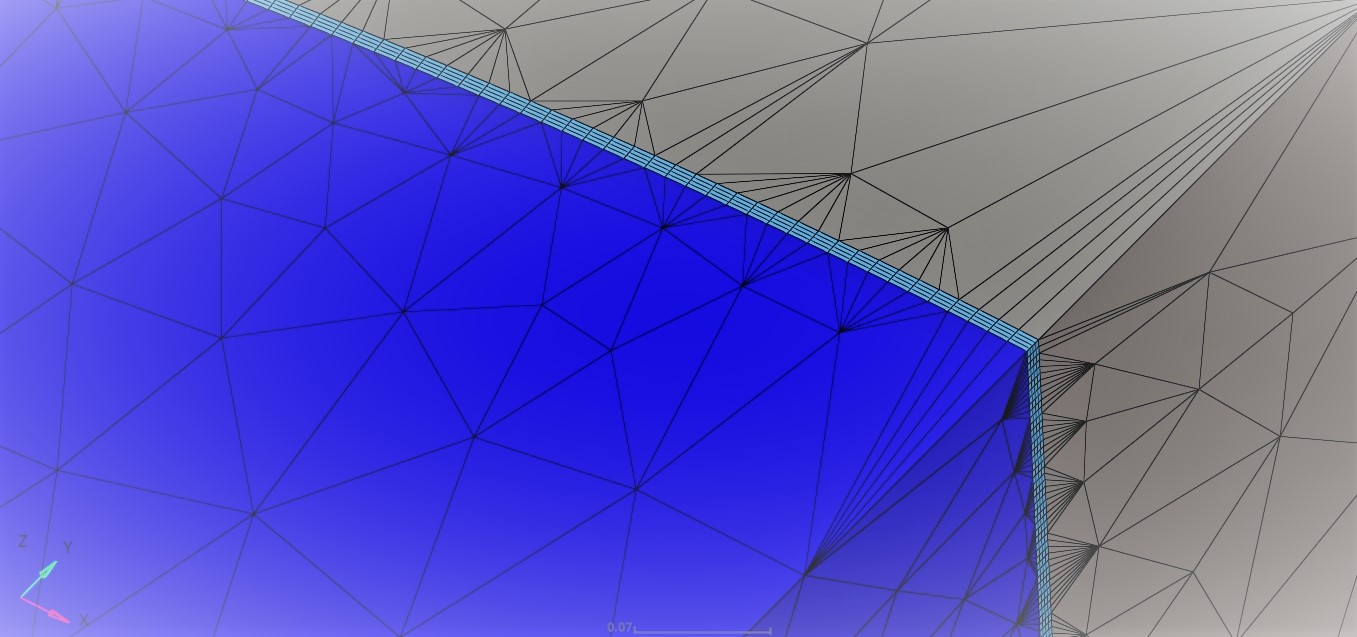# Efficient numerical quadrature for the boundary elements method with application to electrical machinesNowadays, the design with regard to efficiency and reliability is a major task in the development of electrical machines. Therefore, numerical simulations play an increasingly important role.

Due to many favorable properties, the finite element method (FEM) is well established for simulating low-frequency electrical engineering applications, e.g. with nonlinear material characteristics. However, the solution of electromagnetic fields in the air gaps of electrical machines, for example between the stator and the rotor part (as shown here), requires a very fine mesh. That entails a large number of degrees of freedom and thus expensive numerical computations. The coupling of FEM and the boundary element method (BEM) yields an improvement for such situations. BEM is especially used for linear magnetic materials such as air. By coupling of these two methods the air gap doesn’t need to be meshed at all, so a coarser mesh can be used for rotor and stator parts and thus simulation time can be saved. Robert Bosch GmbH uses such a coupling in its in-house simulation software (developed in cooperation with TailSiT) for eddy current problems as they occur in electrical machines.

However, the numerical calculation of the resulting BEM formulation is not trivial, since the Green’s function is numerically integrated on boundary elements. The inverse proportionality of the integrand to the distance between the boundary elements is especially a problem for boundary elements which are close to each other.  Recently, in a master thesis, co-supervised by TU Darmstadt and Robert Bosch GmbH, new approaches were investigated and implemented into the existing in-house code. The calculation of the Gaussian quadrature points by means of variable transformations as well as the use of sparse grids improves the error of the numerical integration by about 2 decimal powers while reducing the computation time of the quadrature by about 15%.</p>